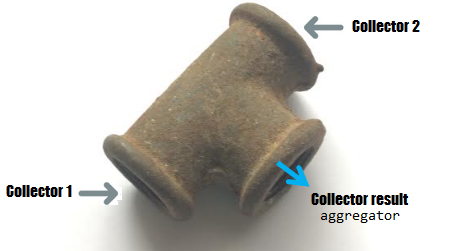# Introducing JDK 12 Teeing

### Tee off with JDK 12!

· Java Zone · Presentation
Save
10.81K ViewsThis article is a brief introduction of the JDK 12 Teeing collector.

You may also like: JDK 12: The Teeing Collector

Starting with JDK 12, we can merge the results of two collectors via `Collectors.teeing()`.The syntax of`teeing()`is:

``````public static <T, R1, R2, R> Collector<T, ?, R> teeing(
Collector<? super T, ?, R1> downstream1,
Collector<? super T, ?, R2> downstream2,
BiFunction<? super R1, ? super R2, R> merger
)``````The result of`teeing()`is a`Collector`that is a composite of two passed downstream collectors. Every element that's passed to the resulting collector is processed by both downstream collectors, and then their results are merged into the final result using the specified`BiFunction`.

Let's take a look at a classical problem. The following class simply maps the number of elements in a stream of integers and their sum:

``````public class CountSum {

private final Long count;
private final Integer sum;

public CountSum(Long count, Integer sum) {
this.count = count;
this.sum = sum;
}
...
}``````

We can obtain this information via`teeing()`, as follows:

``````CountSum countsum = Stream.of(2, 11, 1, 5, 7, 8, 12)
.collect(Collectors.teeing(
counting(),
summingInt(e -> e),
CountSum::new));``````

Here, we have applied two collectors to each element from the stream ( `counting()`
and  `summingInt()` ) and the results have been merged in an instance of `CountSum` :

`CountSum{count=7, sum=46}`

Let's take a look at another problem. This time, the `MinMax` class stores the minimum
and the maximum of a stream of integers:

``````public class MinMax {

private final Integer min;
private final Integer max;

public MinMax(Integer min, Integer max) {
this.min = min;
this.max = max;
}
...
}``````

Now, we can obtain this information, like so:

``````MinMax minmax = Stream.of(2, 11, 1, 5, 7, 8, 12)
.collect(Collectors.teeing(
minBy(Comparator.naturalOrder()),
maxBy(Comparator.naturalOrder()),
(Optional<Integer> a, Optional<Integer> b)
-> new MinMax(a.orElse(Integer.MIN_VALUE),
b.orElse(Integer.MAX_VALUE))));``````

Here, we have applied two collectors to each element from the stream (`minBy()` and
`maxBy()`), and the results have been merged in an instance of `MinMax`:

`MinMax{min=1, max=12}`

Finally, let's consider the following`Melon` class and `List`of `Melon`:

``````public class Melon {

private final String type;
private final int weight;

public Melon(String type, int weight) {
this.type = type;
this.weight = weight;
}
...
}``````
``````List<Melon> melons = Arrays.asList(new Melon("Crenshaw", 1200),
new Melon("Gac", 3000), new Melon("Hemi", 2600),
new Melon("Hemi", 1600), new Melon("Gac", 1200),
new Melon("Apollo", 2600), new Melon("Horned", 1700),
new Melon("Gac", 3000), new Melon("Hemi", 2600)
);``````

The aim here is to compute the total weight of these melons and list their weights. We can map this as follows:

``````public class WeightsAndTotal {

private final int totalWeight;
private final List<Integer> weights;

public WeightsAndTotal(int totalWeight, List<Integer> weights) {
this.totalWeight = totalWeight;
this.weights = weights;
}
...
}``````

The solution to this problem relies on  `Collectors.teeing()` , as follows:

``````WeightsAndTotal weightsAndTotal = melons.stream()
.collect(Collectors.teeing(
summingInt(Melon::getWeight),
mapping(m -> m.getWeight(), toList()),
WeightsAndTotal::new));``````

This time, we have applied the `summingInt()` and `mapping()`collectors. The output is as follows:

``````WeightsAndTotal {
totalWeight = 19500,
weights = [1200, 3000, 2600, 1600, 1200, 2600, 1700, 3000, 2600]
}``````

Done!

The complete source code is available on GitHub.

If you enjoyed this article, then I'm sure you will love my book, Java Coding Problems, which has an entire chapter dedicated to Stream API. Check it out!

## Further Reading

A New JDK 12 Stream API Collection: Collectors#teeing

JDK 12: The Teeing Collector

JDK 12: Merging Collectors and the Challenge of Naming

Topics:
java, streams, collectors, functional programing, jdk 12, teeing

Opinions expressed by DZone contributors are their own.

Comments